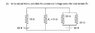# Kirchoff's Laws Two Batteries

• physicsfun_12

## Homework Statement

Hi there, I just want to see if I am doing these questions correctly. Any help with this would be great. The question and circuit diagram are in the attachment. Cheers

## Homework Equations

Kirchoff's Laws for voltage and current. I'm not too sure if there is an equation, you just have to remember that the total current in a node is the same as the input. And for a loop, the total voltage drop = 0.

## The Attempt at a Solution

I said that for loop one, on the left:
60=I120 + I210

For loop two, since the two 20 ohm resistors are effectively one 10 ohm resisitor:
20=I220 + I110

Subing I2 into the first equation, you get an answer of 1.75A for I1

which gives I2=2.5A

Hence a current of 4.25A and voltage of 43.75V

#### Attachments

•Kirchoff's Laws Question.jpg
13.8 KB · Views: 808
Last edited:

Hi physicsfun 12, welcome to PF.

Check your signs. Did you declare which way the current was flowing? Remember that the voltage will be positive if current is flowing into a positive node and negative if flowing into a negative node.

Your equations are very far removed from correct ones. What are I_1 and I_2? There are 3 currents of interest here. Kirchhofs current rule can give you a relation between them. You need to check the signs as well. Since you can combine the two 20 ohm resistances on the right into one 10 ohm resistance, I don't see why you multiply I_1 by 20 since all resistances are 10 ohms.

Your equations are very far removed from correct ones. What are I_1 and I_2? There are 3 currents of interest here. Kirchhofs current rule can give you a relation between them. You need to check the signs as well. Since you can combine the two 20 ohm resistances on the right into one 10 ohm resistance, I don't see why you multiply I_1 by 20 since all resistances are 10 ohms.

Hi there, thnks for your responses.

I multiplied both currents by 20 because i thought there were two currents flowing through the load resisitor since there were two batteries:

My original equations were:
-60 + I110 + (I1 + I2)10 = 0
-20 + I210 + (I1 + I2)10 = 0

I can't see where the third current comes from! Is it the combination of the two?

One last thing, how do you know if a node is a negative or positive node?

First, you correctly simplified the two resistors on the right.

Now to use KCL, the sum of the currents out of a node equals zero. There are three paths out of the top node (Node 1, V1). I'll call these from left to right I1, I2, and I3. They all point down. I'll assign the bottom node as ground.

V1 = I1 R1 + 60,

V1 = I2 R2, (where R2 = RL)

V1 = I3 (R3||R4) + 20, and finally

I1 + I2 + I3 = 0.

That's four equations and four unknowns.

Note that keeping the signs straight is important. I haven't solved this, but it looks like current will be flowing into the 20V battery rather than out of it.

I have tried the question again and this time got Current=2.666A and Voltage=26.66V

Do you know if these answers are correct?

Thanks again

They seem right.

I just did this and get the same answer.

WOO! Been trying for ages thanks everyone.

Take care